# Area + fractions - math problems

#### Number of problems found: 82

• DivideDivide area of rectangles with dimensions 32m and 10m by the ratio 7: 9. What area corresponds to a smaller section?
• GardenThe rectangular garden has dimensions of 27 m and 30 m. Peter and Katka split it in a ratio of 4:5. How many square meters did Katkin measure part of the garden?
• MO Z9–I–2 - 2017In the VODY trapezoid, VO is a longer base and the diagonal intersection K divides the VD line in a 3:2 ratio. The area of the KOV triangle is 13.5 cm2. Find the area of the entire trapezoid.
• Three shapes1/5 of a circle is shaded. The ratio of area if square to the sum of area of rectangle and that of the circle is 1:2. 60% of the square is shaded and 1/3 of the rectangle is shaded. What is the ratio of the area of circle to that of the rectangle?
• Magic belt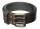The magic rectangular belt has the property that whenever its owner wants something, the length of the belt is reduced to 1/2 and the width to 1/3. After three such wishes, the belt had a content area 4 cm2. What was its original length if the original wi
• Trapezoid thirdsThe ABCD trapezoid with the parallel sides of the AB and the CD and the E point of the AB side. The segment DE divides the trapezoid into two parts with the same area. Find the length of the AE line segment.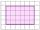Shade the area on the grid that shows 5/8 x 2/4
• Hamster cage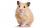Ryan keeps his hamster cage on his dresser. The area of the top of Ryan's dresser is 1 2\3 as large as the area of the bottom of his hamster cage. The area of the dresser top is 960 square inches. How many square inches of his dresser top are not covered
• Above EarthTo what height must a boy be raised above the earth to see one-fifth of its surface.
• Land areaThe land area of Asia and Africa are in a 3: 2 ratio, the European and African are is 1:3. What are the proportions of Asia, Africa, and Europe?
• Mr. Happy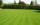Mr. Happy planted 36.6 meters square gardens grass; It's a third of the garden more than half of the garden. What is square area of this garden?
• Oceans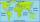The Earth's surface is approximately 510,000,000 km2 and is 7/10 covered by oceans. Of which 1/2 covers the Pacific Ocean, the Atlantic Ocean 1/4, the Indian Ocean 1/5 and the Arctic Ocean 1/20. What parts of the Earth's surface cover each ocean?
• Garden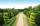The garden has a rectangular shape with lengths of 25 and 40 meters. It has been expanded so that each of its size increased by one fifth. How many square meters increased its acreage?
• Cuboid boxHow many m2 paper is needed for the sticking cuboid box of dimensions 50 cm, 40 cm and 30 cm? To the folds add one-tenth the area.
• Mystery of stereometrieTwo regular tetrahedrons have surfaces 88 cm2 and 198 cm2. In what ratio is their volumes? Write as a fraction and as a decimal rounded to 4 decimal places.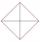In the square ABCD point P is in the middle of the DC side and point Q in the middle pages AD. If the area of quadrilateral BQPC is 49 cm2, what is the area of ABCD?
• ConeCircular cone of height 15 cm and volume 5699 cm3 is at one-third of the height (measured from the bottom) cut by a plane parallel to the base. Calculate the radius and circumference of the circular cut.
• Trapezoid MOThe rectangular trapezoid ABCD with the right angle at point B, |AC| = 12, |CD| = 8, diagonals are perpendicular to each other. Calculate the perimeter and area of ​​the trapezoid.
• Cone A2VThe surface of the cone in the plane is a circular arc with central angle of 126° and area 415 cm2. Calculate the volume of a cone.
• FloorThe floor area of ​​the room is 31 m2 and has a width of 4.3 m. How many centimeters of circumference measured the floor on the map at the scale 1:75?

Do you have an exciting math question or word problem that you can't solve? Ask a question or post a math problem, and we can try to solve it.

We will send a solution to your e-mail address. Solved examples are also published here. Please enter the e-mail correctly and check whether you don't have a full mailbox.

Need help to calculate sum, simplify or multiply fractions? Try our fraction calculator. Area - math problems. Fraction Word Problems.